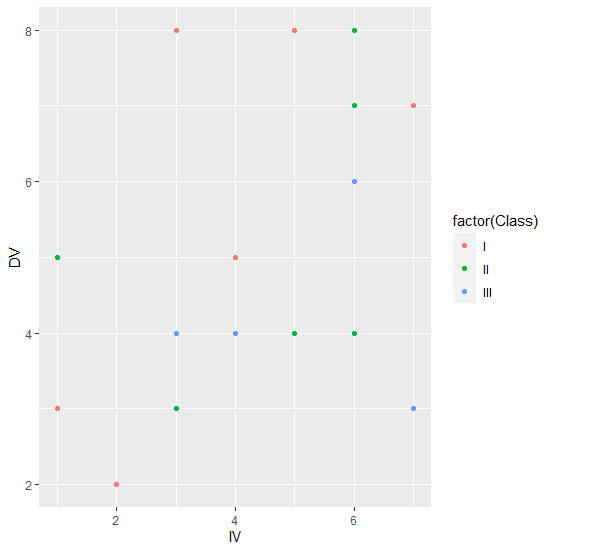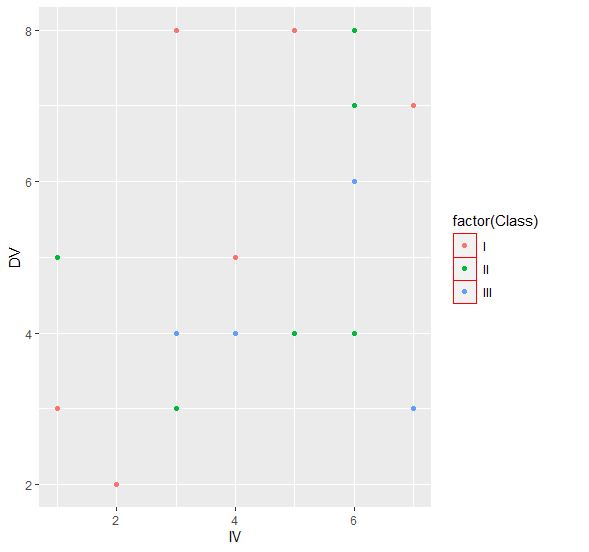# Change the color of legend element border using ggplot2 in R.

R ProgrammingServer Side ProgrammingProgramming

To change the color legend element border using ggplot2, we can use theme function where can put color in legend.key argument to desired color with element_rect.

For Example, if we have a data frame called df that contains three columns say X and Y and F where X and Y are numerical and F is categorical then we can create scatterplot between X and Y with blue color of legend element border by using the command given below −

ggplot(df,aes(IV,DV))+geom_point(aes(colour=factor(Class)))+theme(legend.key=element_rect(colour="red"))

## Example

Following snippet creates a sample data frame −

IV<-rpois(20,5)
DV<-rpois(20,5)
Class<-sample(c("I","II","III"),20,replace=TRUE)
df<-data.frame(IV,DV,Class)
df

The following dataframe is created

  IV DV Class
1 4 4   III
2 4 5   III
3 3 4   I
4 3 8   I
5 3 3   II
6 7 7   I
7 5 4   II
8 3 4   III
9 1 5   II
10 6 8   II
11 3 3   II
12 4 5   II
13 5 8   I
14 2 2   I
15 6 6   III
16 6 4   II
17 1 3   I
18 4 5   I
19 6 7   II
20 7 3   III

To load ggplot2 package and create scatterplot between IV and DV with points color based on values in Class on the above created data frame, add the following code to the above snippet −

IV<-rpois(20,5)
DV<-rpois(20,5)
Class<-sample(c("I","II","III"),20,replace=TRUE)
df<-data.frame(IV,DV,Class)
library(ggplot2)
ggplot(df,aes(IV,DV))+geom_point(aes(colour=factor(Class)))

## Output

If you execute all the above given snippets as a single program, it generates the following Output −To create scatterplot between IV and DV with points color based on values in Class having legend borders in red on the above created data frame, add the following code to the above snippet −

IV<-rpois(20,5)
DV<-rpois(20,5)
Class<-sample(c("I","II","III"),20,replace=TRUE)
df<-data.frame(IV,DV,Class)
library(ggplot2)
ggplot(df,aes(IV,DV))+geom_point(aes(colour=factor(Class)))+theme(legend.key=element_rect(colour="red"))

## Output

If you execute all the above given snippets as a single program, it generates the following Output −